Browse by Topic

# A Mathematical Modeling Approach to Monitoring Liver Function in Drug Trials

Explore the contents of this article with a free Wolfram System Modeler trial. Mathematical modeling is not just used for understanding and designing new products and drugs; modeling can also be used in health care, and in the future, your doctor might examine your liver with a mathematical model just like the one researchers at AstraZeneca have developed.The liver is a vital organ, and currently there isn’t really a way to compensate for loss of liver function in the long term. The liver performs a wide range of functions, including detoxification, protein synthesis, and secretion of compounds necessary for digestion, just to mention a few. In the US and Europe, up to 15 % of all acute liver failure cases are due to drug-induced liver injury, and the risk of injuring the liver is of major concern in testing new drug candidates. So in order to safely monitor the impact of a new drug candidate on the liver, researchers at the pharmaceutical company AstraZeneca have recently published a method for evaluating liver function that combines magnetic resonance imaging (MRI) and mathematical modeling—potentially allowing for early identification of any reduced liver function in humans.

Last year, Wolfram MathCore and AstraZeneca worked together on a project where we investigated some modifications of AstraZeneca’s modeling framework. We presented the promising results at the ISMRM-ESMRMB Joint Annual Meeting, which is the major international magnetic resonance conference. In this blog post, I’ll show how the Wolfram Language was used to calculate liver function and how more complex models of liver function can be implemented in Wolfram System Modeler.

A quick introduction to the method

You might be wondering what happens within the liver during the examination using a mathematical model. It all starts after the injection of the MRI contrast agent into the blood, where it spreads and ultimately reaches the liver. Inside the liver (see the figure below) the blood vessel walls are highly permeable, like a coffee filter, allowing for a rapid diffusion of the agent into the extracellular space. The MRI contrast agent accumulates in the liver cells, and finally is excreted into the bile. The accumulation and efflux require that the cells are healthy, have enough energy, and are not overloaded with other work. If the cells are compromised, the transfer rates of the agent will be reduced. A reduced liver function can thus be observed by the calculated transfer rates in the model.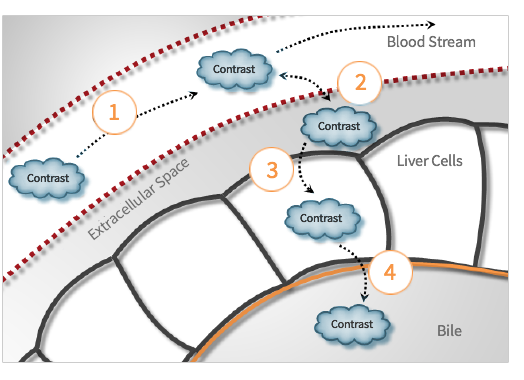Okay, now that you have some background on the basics of how liver function can be estimated, let’s move on to the fun part of computation and modeling. I will start by showing examples of the types of data we use.

Extracting data

Data is extracted from the images in regions of interest (ROIs) within the liver as well as the spleen. The latter is used as a good and stable surrogate for measuring the amount of contrast agent within the blood directly, since the splenic cells do not accumulate any contrast agent; this means that our measurement in the spleen is only influenced by the contrast agent in the spleen’s blood vessels. The ROIs can be of any geometry. In Mathematica, I draw and modify ROIs with a custom interactive interface. Of course, you could also select the entire liver or other distinct parts in images using some of the automated algorithms implemented in Mathematica.

Below is an example of the types of images that we used. These two images were acquired about five minutes after the injection of the contrast agent, which is the reason that the liver is so bright (compared to, for instance, the muscles that can be seen on the sides of the images). The images are captured on a coronal imaging plane, which means that the images are what you see when the subject is lying down on its back and you are looking down on the subject from above. Images a) and b) are at different heights from the table, where b) is further from the table; there you can also see a portion of the spleen.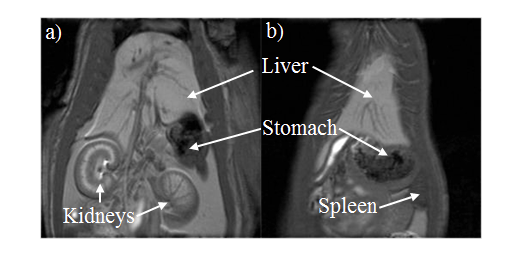If you are familiar with human anatomy, especially in medical imaging, you might have noticed that the images don’t look like the inside of a human. Well, in that case you are right: the images show the inside of a rat, which were the subjects used in the study performed at AstraZeneca.

Data

AstraZeneca has gathered quite a lot of high-quality data on its rats, using the approach mentioned above, and I will not show all of that here. Rather, I’ve been inspired by early TV chefs and prepared some of the data. I will now exemplify the method with three subjects; i) a rat with normal liver function, ii) a rat with slightly reduced liver function, and iii) a rat with severely reduced liver function. The data covers 60 minutes, where the first four minutes are baseline (prior to the injection of the contrast agent) and used for the post-processing, so those values should by definition be equal to zero.

As I mentioned previously, data is extracted from the image series in two different regions. One of these two regions is the liver, and after some post-processing of this data, we get the mean contrast agent concentrations within the liver cells (I will name this data set cHep in the code from here on). You can see what these concentration time series look like for all three subjects in the figure below. I will use this data for model fitting.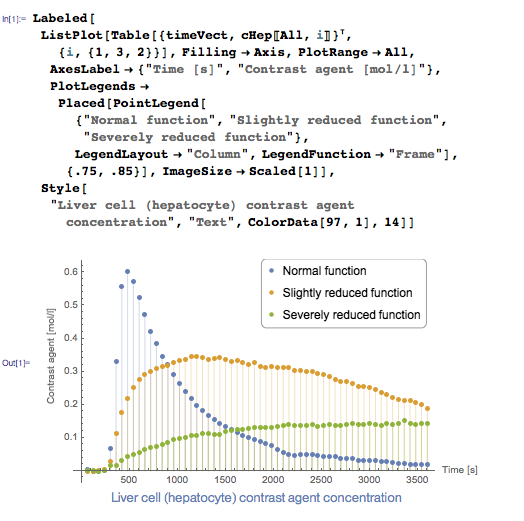The second region from which data is extracted is the spleen, and after the post-processing, we get the mean contrast agent concentration within the extracellular space (I will name this data set cES). This data tells us how much contrast agent is available for accumulation in the liver, and it will be used as input in the models. You can see what this data looks like for all three subjects in the figure below.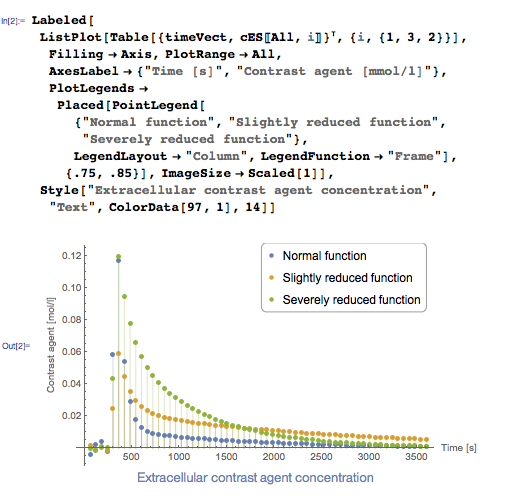In order to use the measured extracellular concentrations (cES) in the model, the values need to be continuous. So let’s go ahead and generate an interpolating function (intES) based on these values, one for each case. Since there is no contrast in the first four minutes, by experimental design, we set these to zero.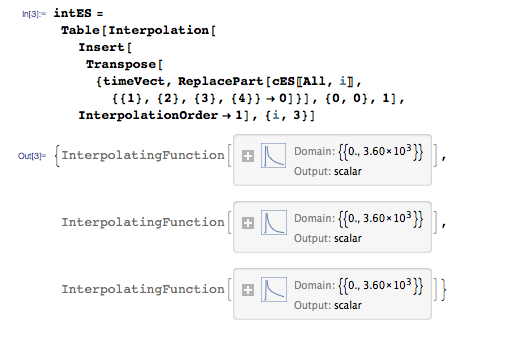And we can check the agreement with the data. Here I’ll just show the normal case, remembering that we just set the first four points to be identical to zero.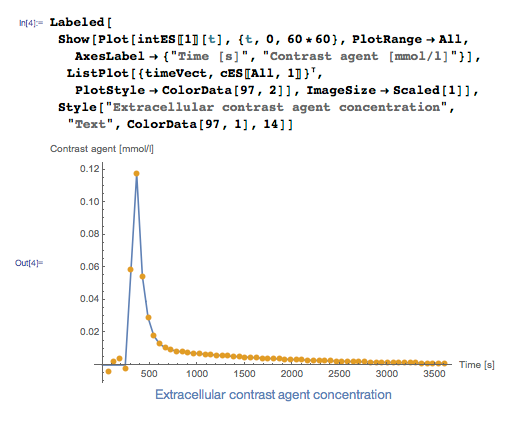Defining the model

The model is defined using an ordinary differential equation, where we solve for the concentration of the contrast agent within the liver cells. The uptake comes from the extracellular space (step 3 in the figure) governed by the kinetic parameter k1. The transfer of this agent into the bile is described using Michaelis–Menten kinetics (step 4 in the figure) using the kinetic parameters Vmax and Km.With the initial condition for the concentration in liver cells being: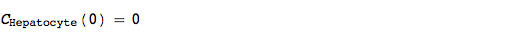In the project, a simplification of the above model was investigated, specifically, the efflux into the bile was described with a linear rate equation. Since Michaelis–Menten kinetics are approximately linear in low substrate concentrations, this simplification can be valid if the concentrations of the contrast agent are low enough.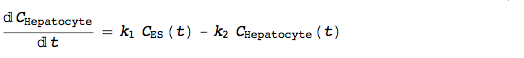Now it’s time to solve the two models using ParametricNDSolve. Since we have the interpolating functions (intES), specific for each subject, inside the model, we need to compute a solution specifically for each subject: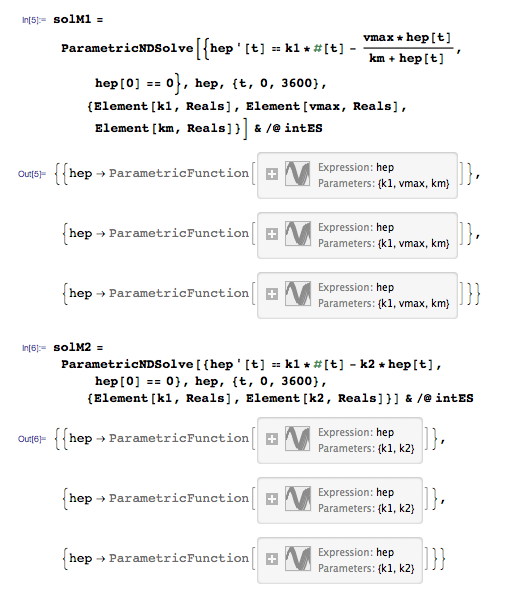Fitting the model to data

In order to fit the model to the data, we need a target or objective function to guide our optimization algorithm in the correct direction. In this case, I’ve used the Euclidean norm, as a measure of the goodness of model fit: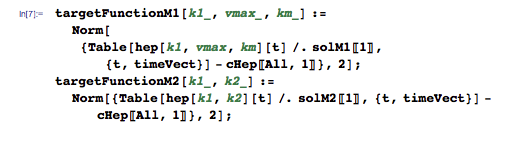Whenever I use a global optimization algorithm for estimating the parameters, which takes a fair bit of time to complete, I like to see where the algorithm is moving in the parameter space. This way, I can see if it struggles, or maybe it finds a local minima it can’t get out of, or anything else that might be fun and educational to observe. For this purpose, the monitor functionalities are suitable: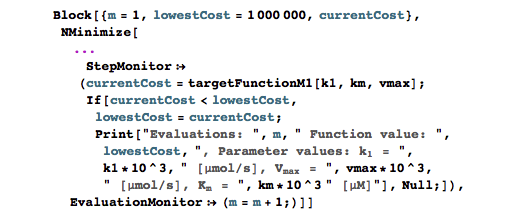In order to improve the optimization, we also include a list of reasonable parameter boundaries and start guesses, which covers a wide range of scenarios.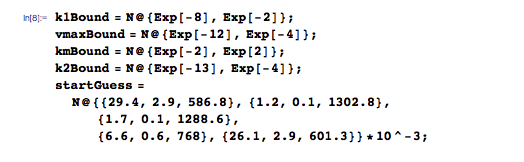Completing the code for the above Block, with the necessary inputs to NMinimize, we get the following compact piece of code that helps us with the answer to: How good is the liver function?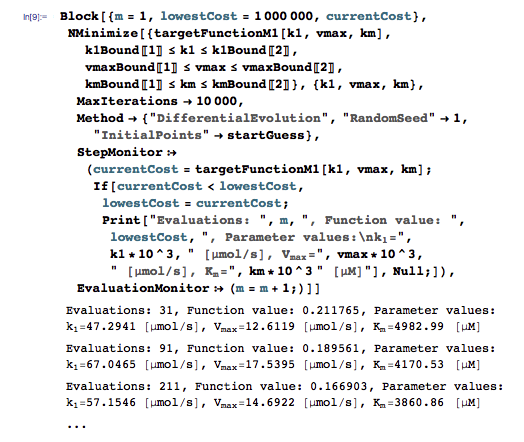If you’re interested in the different global optimization schemes available in Mathematica, there is great tutorial available here.

Once again the TV-chef magic kicks in, and we have a bunch of optimal parameter values already prepared for the three subjects (for both models):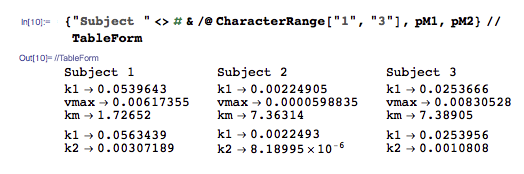And we combine the parameter values with the parametric solutions of the models to calculate the model predictions for both models and all three cases: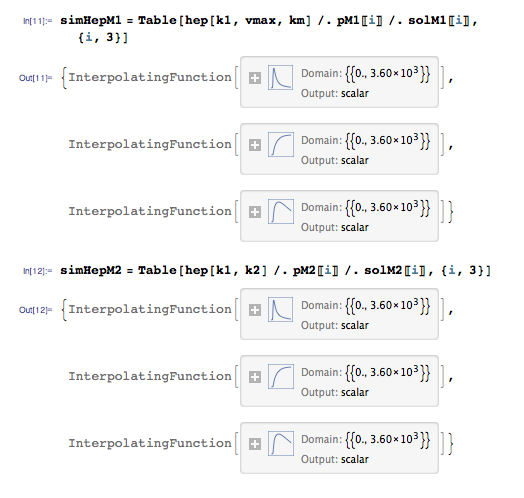Results

Below you can see the predictions made by the model with the fitted parameter values compared to the data on our three subjects, ranging from normal to severely reduced liver function.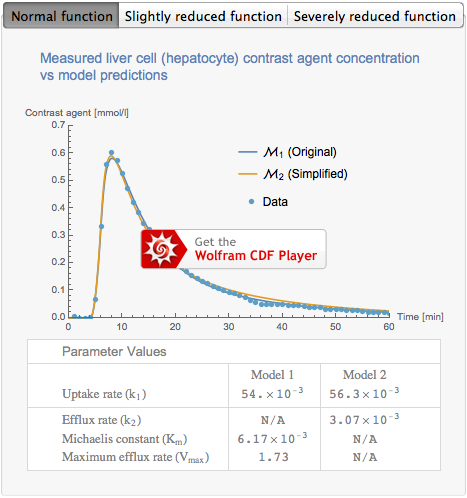As you can see in the figures, there is a clearly reduced concentration of contrast in the last case. This reduction can be appreciated quantitatively in the table underneath the figures, where the uptake rate is almost a factor 20 lower in the last case. It’s noteworthy that the uptake rate is in all practical aspects identical for both model variants in the three cases, indicating that the use of a linear description of the efflux of contrast into the bile instead of Michaelis–Menten kinetics might be valid. Also, the models are able to predict the data very well; of course, you wouldn’t be reading this unless that was the case (data from humans is much noisier, for various reasons).

In the animation below, I’ve correlated the model predictions for the rat with normal liver function with the acquired images, so that you might better appreciate how the numbers relate to the images. As you might remember from the beginning of this post, the liver is the large organ at the top of the images.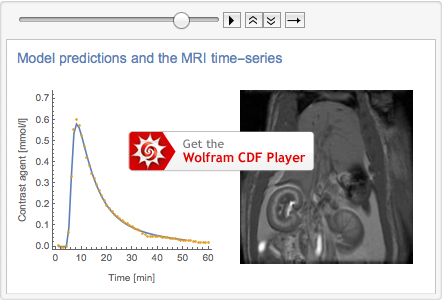On the horizon

In the original paper by Jose Ulloa et al., where the first model and the data come from, the model parameters were able to separate between the different groups with strong significance. In this project we found that the uptake rate was in practice identical for both model variants, and that the simplified model was also good at separating between the different groups.

These methods that AstraZeneca has developed were evaluated on rats, and the work continues at AstraZeneca, and in other pharmaceutical companies, on refining and ultimately utilizing these methods for investigating liver function in pre-clinical and clinical trials, as well as in the clinic. We are all very excited about these results, and as you read this, both AstraZeneca and Wolfram MathCore are involved in new projects dedicated to evaluating these methodologies further, even applying them to patients suffering from liver disease.

Modeling liver function in Wolfram System Modeler

In the above calculations, I used Mathematica exclusively; however these models can just as easily be implemented in System Modeler by using the BioChem library, as shown by the figure below. In this particular case, the model contains so few states that the model implementation is just as fast programmatically in Mathematica, but if this were a larger or hierarchical model, System Modeler would be my first choice. It’s also worth noting that it if I had implemented the model using System Modeler, the code for fitting the parameters would have been basically the same. In principle, I would only need to modify to the target function.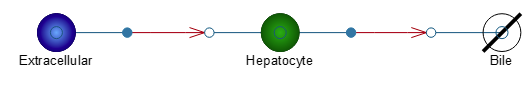Wolfram MathCore collaborates with researchers at Linköping University’s Center for Medical Image Science and Visualization (CMIV) on research aimed toward a comprehensive non-invasive diagnostic MRI-based toolset for patients suffering from liver disease. The collaboration has for example led to the development of a mathematical model for estimating liver function in humans based on, in principle, the same kind of MRI data we have shown in this post. The underlying assumptions for this model and the one used above are very similar. The figure below shows this model implemented in System Modeler using the BioChem library, and more details on this model can be found on our example pages.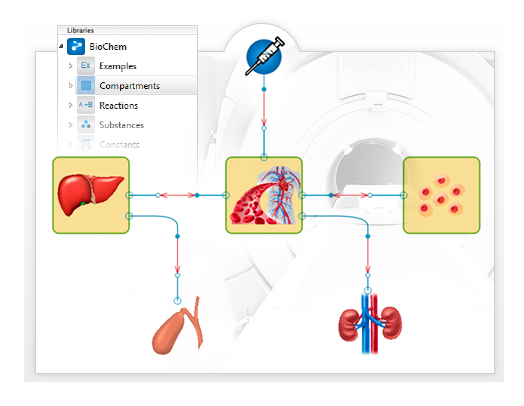If you want to try the tools I’ve used for yourself, you can get a trial of both Mathematica and System Modeler and get cracking.

At Wolfram MathCore, we have done numerous consultancy projects for a wide range of customers, from machine dynamics and 3D mechanical systems to thermodynamics and, of course, life science. The results from another life science project we worked on together with MedImmune (a subsidiary of AstraZeneca) were recently published in a daughter journal of Nature. So, if you need to solve tricky problems or want to get your modeling and simulation project up and running quickly with our tools, don’t hesitate to contact us at Wolfram MathCore!